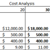## Project ManagementNet Present Value (NPV) Analysis The students were asked to perform a financial analysis for a specific project. The projected costs and benefits were spread over four years, with a discount rate of 9%. The students had to calculate the NPV, ROI, and the year in which payback would occur. NPV AnalysisCost Analysis The students were asked to perform a cost analysis, and answer questions based on given figures. They also had to determine the costs for different amounts of attendees, along with specific costs such as rent, mailouts, and handouts for a two day course. Cost Analysis